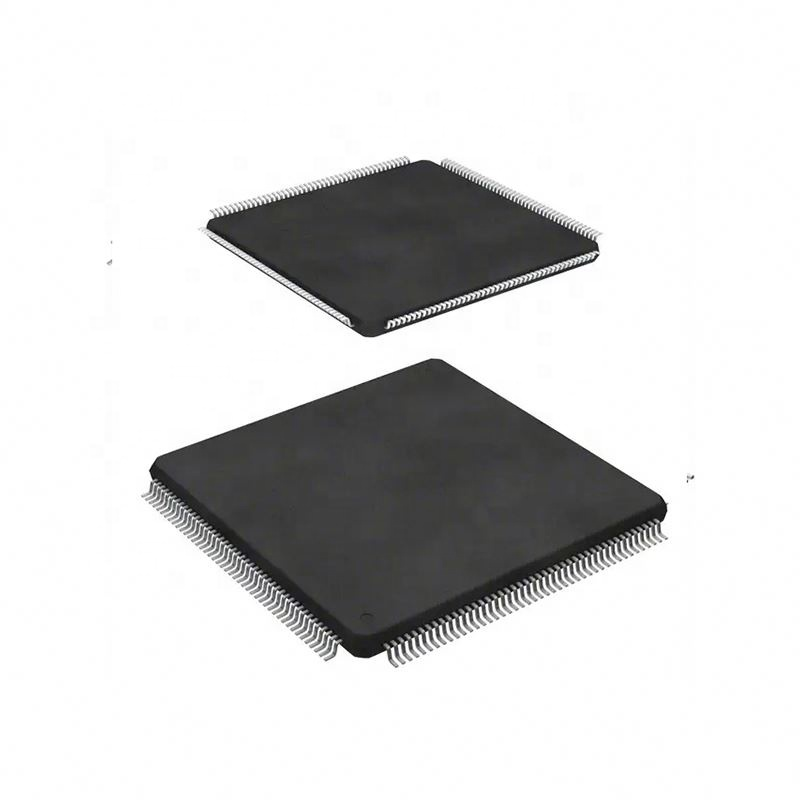< img src="https://mc.yandex.ru/watch/53493796" style="position:absolute; left:-9999px;" alt="" />
Need Help?## ATMEGA64A-AU IC Electronic Components Integrated Circuits IC

• Model Number: ATMEGA64A-AU
• Type: integrated circuit
• Brand Name: Original
• D/C: 2021+
• Voltage – Breakdown: Standard
• Voltage – Isolation: Standard
• Current – Output High, Low: Standard
• Current – Peak Output: Standard
• Voltage – Forward (Vf) (Typ): Standard

## Description

• Current – DC Forward (If) (Max): Standard
• Input Type: Standard
• Output Type: Standard
• Current Transfer Ratio (Min): Standard
• Current Transfer Ratio (Max): Standard
• Voltage – Output (Max): Standard
• Voltage – Off State: Standard
• Static dV/dt (Min): Standard
• Current – LED Trigger (Ift) (Max): Standard
• Current – On State (It (RMS)) (Max): Standard
• Impedance: Standard
• Impedance – Unbalanced/Balanced: Standard
• LO Frequency: Standard
• RF Frequency: Standard
• Input Range: Standard
• Output Power: Standard
• Frequency Bands (Low / High): Standard
SEND EMAIL TO US